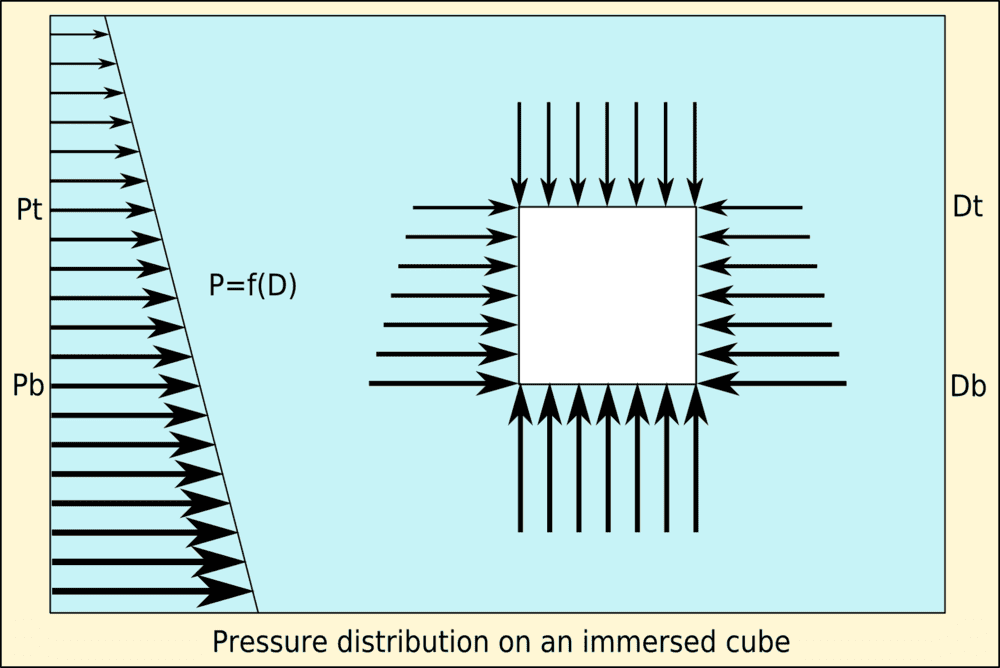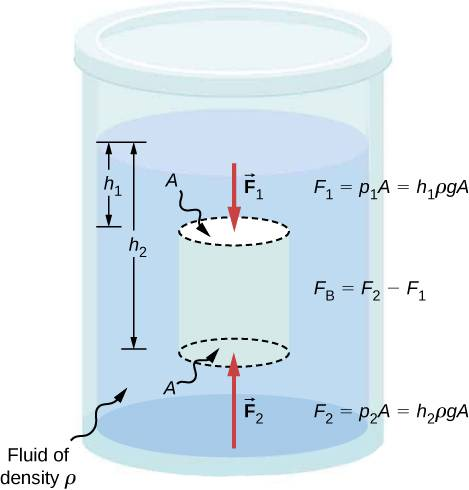# Weight of fluids on a submerged person?

• aspodkfpo

#### aspodkfpo

Pg 9 Q 11 A)

Was wondering why there isn't a weight of water in the force diagram? The boy is submerged, as I understand.

Do we normally include the weight of a fluid on someone or how does this work?
For instance, is there a weight of air on us when we stand on land? What is this quantity of air? Is it a negligible amount that collides with our head at any given time, or a rectangular prism of air stretching from the surface of our head to the atmosphere?

"Draw a free body diagram showing the forces acting on the boy when his lungs are empty after breathing out slowly. Indicate the magnitude of the forces acting on the boy by the size of the arrows you draw to represent the forces."

We should only be analyzing the external forces acting on the body of the boy.

https://www.physicsforums.com/media/free-body-diagrams.98/

https://en.m.wikipedia.org/wiki/Free_body_diagram

https://en.m.wikipedia.org/wiki/Buoyancy

The substances inside the body are not important, only its average density, which interacts with the density of the fluid around it.
More or less air in his lungs means less or more dense body, which affects the buoyancy effect.

Last edited:
Do we normally include the weight of a fluid on someone or how does this work?
No. Fluids can flow sideways, so there is no simple relationship between the weight of a parcel of fluid and the force exerted on an object directly below. Instead, think in terms of pressure.
As this is a buoyancy question, the easiest way is to use Archimedes' principle. This avoids having to integrate the pressure over the surface area of the body. For more details see https://www.physicsforums.com/insights/frequently-made-errors-mechanics-hydrostatics/.

•aspodkfpo
"Draw a free body diagram showing the forces acting on the boy when his lungs are empty after breathing out slowly. Indicate the magnitude of the forces acting on the boy by the size of the arrows you draw to represent the forces."

We should only be analyzing the external forces acting on the body of the boy.

https://www.physicsforums.com/media/free-body-diagrams.98/

https://en.m.wikipedia.org/wiki/Free_body_diagram

https://en.m.wikipedia.org/wiki/Buoyancy

The substances inside the body are not important, only its average density, which interacts with the density of the fluid around it.
More or less air in his lungs means less or more dense body, which affects the buoyancy effect.
Was referring to the weight of the water on top of his body, because he is submerged (i.e. below water level).

Archimedes principle still holds true. Yes there is greater pressure on the "top" as one submerges, but there is also greater pressure on the "bottom". The net force is still Archimedes buoyancy.

•Lnewqban
Archimedes principle still holds true. Yes there is greater pressure on the "top" as one submerges, but there is also greater pressure on the "bottom". The net force is still Archimedes buoyancy.

So we don't consider the weight of water, but look at is as buoyancy?

So we don't consider the weight of water, but look at is as buoyancy?
Yes.

How does one find buoyancy (or more precisely buoyant force)? It depends upon the weight of water displaced...which is related to the difference in pressure "below" and "above". So yes...

•Lnewqban and aspodkfpo
Was referring to the weight of the water on top of his body, because he is submerged (i.e. below water level).
My apologies, I misread your post.Copied from the third link of my previous post:

"Consider a cube immersed in a fluid with the upper surface horizontal.

The sides are identical in area, and have the same depth distribution, therefore they also have the same pressure distribution, and consequently the same total force resulting from hydrostatic pressure, exerted perpendicular to the plane of the surface of each side.

There are two pairs of opposing sides, therefore the resultant horizontal forces balance in both orthogonal directions, and the resultant force is zero.

The upward force on the cube is the pressure on the bottom surface integrated over its area. The surface is at constant depth, so the pressure is constant. Therefore, the integral of the pressure over the area of the horizontal bottom surface of the cube is the hydrostatic pressure at that depth multiplied by the area of the bottom surface.

Similarly, the downward force on the cube is the pressure on the top surface integrated over its area. The surface is at constant depth, so the pressure is constant. Therefore, the integral of the pressure over the area of the horizontal top surface of the cube is the hydrostatic pressure at that depth multiplied by the area of the top surface.
...
An object of any shape can be approximated as a group of cubes in contact with each other, and as the size of the cube is decreased, the precision of the approximation increases. The limiting case for infinitely small cubes is the exact equivalence.

Angled surfaces do not nullify the analogy as the resultant force can be split into orthogonal components and each dealt with in the same way."•hutchphd